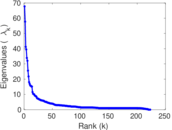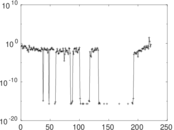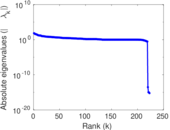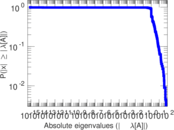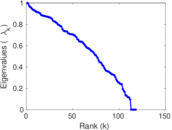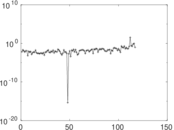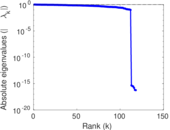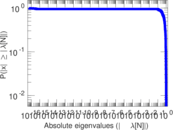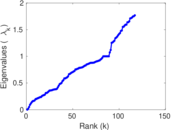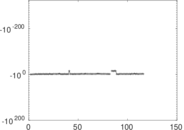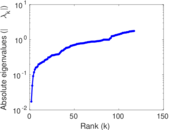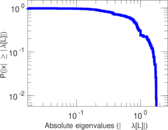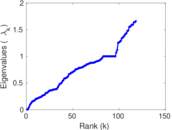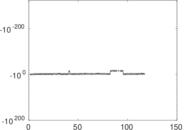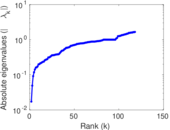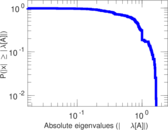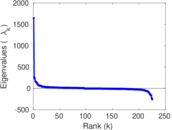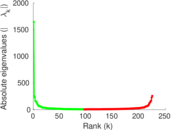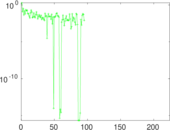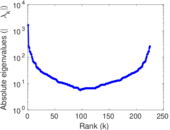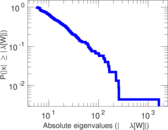# Wikiquote edits (be)

This is the bipartite edit network of the Belarusian Wikiquote. It contains users and pages from the Belarusian Wikiquote, connected by edit events. Each edge represents an edit. The dataset includes the timestamp of each edit.

 Code `qbe` Internal name `edit-bewikiquote` Name Wikiquote edits (be) Data source http://dumps.wikimedia.org/ AvailabilityDataset is available for download Consistency checkDataset passed all tests Category Authorship network Dataset timestamp 2017-10-20 Node meaning User, article Edge meaning Edit Network formatBipartite, undirected Edge typeUnweighted, multiple edges Temporal dataEdges are annotated with timestamps

## Statistics

 Size n = 1,658 Left size n1 = 225 Right size n2 = 1,433 Volume m = 4,036 Unique edge count m̿ = 2,409 Wedge count s = 172,393 Claw count z = 15,234,971 Cross count x = 1,218,535,506 Square count q = 14,696 4-Tour count T4 = 812,826 Maximum degree dmax = 838 Maximum left degree d1max = 838 Maximum right degree d2max = 48 Average degree d = 4.868 52 Average left degree d1 = 17.937 8 Average right degree d2 = 2.816 47 Fill p = 0.007 471 51 Average edge multiplicity m̃ = 1.675 38 Size of LCC N = 1,369 Diameter δ = 12 50-Percentile effective diameter δ0.5 = 3.462 07 90-Percentile effective diameter δ0.9 = 5.395 83 Median distance δM = 4 Mean distance δm = 3.947 07 Gini coefficient G = 0.712 987 Balanced inequality ratio P = 0.216 799 Left balanced inequality ratio P1 = 0.136 274 Right balanced inequality ratio P2 = 0.302 032 Relative edge distribution entropy Her = 0.813 942 Power law exponent γ = 3.488 82 Tail power law exponent γt = 2.241 00 Tail power law exponent with p γ3 = 2.241 00 p-value p = 0.000 00 Left tail power law exponent with p γ3,1 = 1.731 00 Left p-value p1 = 0.540 000 Right tail power law exponent with p γ3,2 = 2.951 00 Right p-value p2 = 0.071 000 0 Degree assortativity ρ = −0.308 670 Degree assortativity p-value pρ = 2.434 10 × 10−54 Spectral norm α = 67.768 4 Algebraic connectivity a = 0.017 280 5 Spectral separation |λ1[A] / λ2[A]| = 1.173 75 Controllability C = 1,214 Relative controllability Cr = 0.734 867

## Plots

### Fruchterman–Reingold graph drawing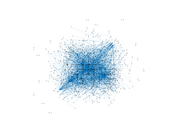### Degree distribution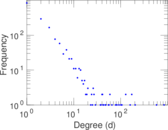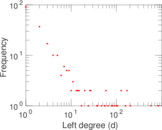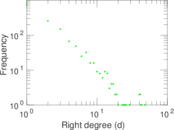### Cumulative degree distribution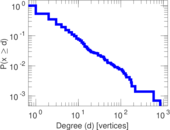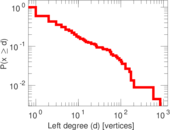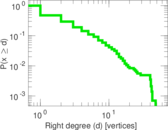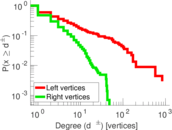### Lorenz curve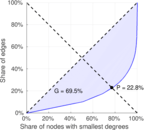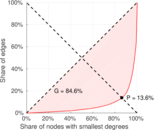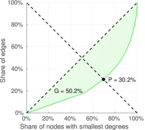### Spectral distribution of the adjacency matrix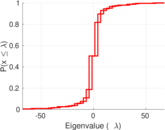### Spectral distribution of the normalized adjacency matrix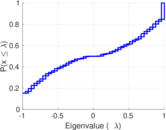### Spectral distribution of the Laplacian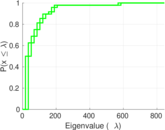### Spectral graph drawing based on the adjacency matrix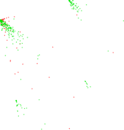### Spectral graph drawing based on the Laplacian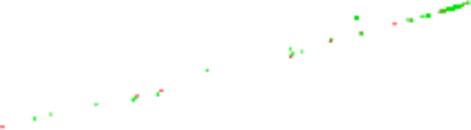### Spectral graph drawing based on the normalized adjacency matrix### Degree assortativity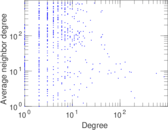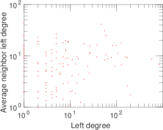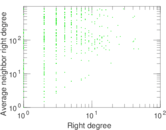### Zipf plot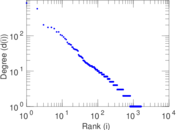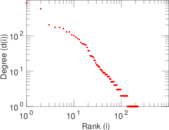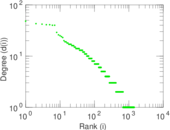### Hop distribution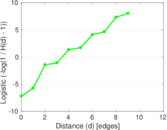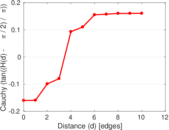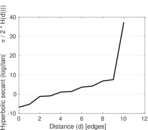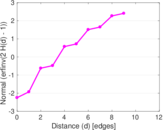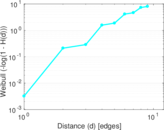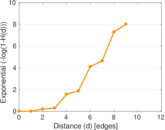### Double Laplacian graph drawing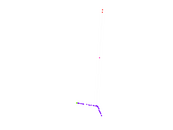### Delaunay graph drawing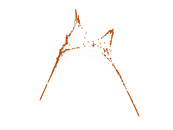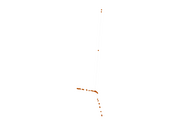### Edge weight/multiplicity distribution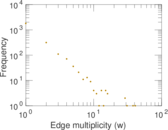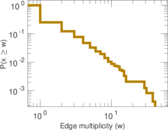### Temporal distribution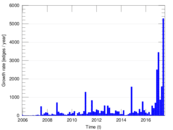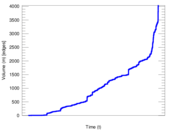### Temporal hop distribution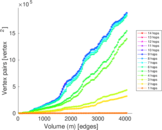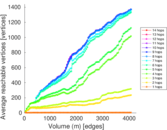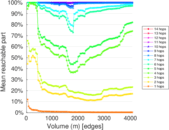### Diameter/density evolution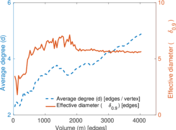### Matrix decompositions plots